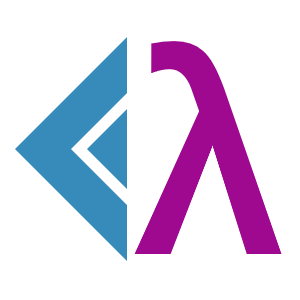#LambdaCalculus 1.0.0-lambda

This is a prerelease version of LambdaCalculus.
`Install-Package LambdaCalculus -Version 1.0.0-lambda`
`dotnet add package LambdaCalculus --version 1.0.0-lambda`
`<PackageReference Include="LambdaCalculus" Version="1.0.0-lambda" />`
For projects that support PackageReference, copy this XML node into the project file to reference the package.
`paket add LambdaCalculus --version 1.0.0-lambda`
`#r "nuget: LambdaCalculus, 1.0.0-lambda"`
#r directive can be used in F# Interactive, C# scripting and .NET Interactive. Copy this into the interactive tool or source code of the script to reference the package.
```// Install LambdaCalculus as a Cake Addin

// Install LambdaCalculus as a Cake Tool
#tool nuget:?package=LambdaCalculus&version=1.0.0-lambda&prerelease```

## λ calculus in F#

Fun library for lambda calculus made purely in F#. It has a web app made for demonstration.

### Syntax of λ-calculus

Rules:

• Variables are single-character lower-case Latin letters.
• Lambda starts with `\`, then is followed by 1 or more variable, then a dot (`.`), then expression. Until wrapped with parentheses, the body of a lambda has a lower priority than `()` (e. g. `\x.(x)(y)` is the same as `(\x.x)(y)`).
• Associativity is left-sided: `xyz` is `(xy)z`.
• A nested lambda can be shortcuted: `\x.\y.\z.xyz` is the same as `\xyz.xyz`.
• Symbol `λ` can be used instead of `\`, but is not essential.

Examples:

• `x` - an expression of one free variable x
• `xy` - `y` applied to `x`
• `\x.x` - an identity
• `\x.xx` - a lambda which returns its only parameter applied to itself
• `(\x.x)y` - `y` applied to identity (will return `y` after beta reduction)
• `(\x.xx)(\x.xx)` - simple example of beta-irreducible expression

### Library API

Expression is defined as following:

``````type Expression =
| Variable of char
| Lambda of Head : Variable * Body : Expression
| Applied of Lambda : Expression * Argument : Expression
``````

in `LambdaCalculus.Atoms`.

In the same module `LambdaCalculus.Atoms` there are

• `betaReduce` or `βReduce`: `Expression -> Expression` performs beta-reduction, that is, simplification of an expression (starting from bottom, replaces parameters of lambdas in their bodies with the applied expressions)
• `alphaEqual` or `αEqual`: `Expression -> Expression -> Expression` compares two expressions up to lambdas parameter names
• `substitute`: `char -> Expression -> Expression -> Expression` replaces the given variable with a given expression. In case of conflicts between it and a local variable in a lambda, it will alpha-convert the lambda's parameter to a new name.

In the module `LambdaCalculus.Parsing` there is `parse` which takes a string as an argument, and returns a `Result` of `Expression` and `string`.

### Fun facts

The library is made in pure functional programming. Even the parser has no exceptions, early returns, for-loops. The logic is implemented with recursion, pattern matching, and computation expressions.

### NuGet packages

This package is not used by any NuGet packages.

### GitHub repositories

This package is not used by any popular GitHub repositories.